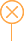语言选择

400-629-1299
En

# 浅析啤酒糟干燥前后的重量变化

###### 返回列表作者：鼎力烘干机生产厂家 发表日期：2019-09-18

• 干基啤酒糟

• 干麦糟量

G 1 =3200 ⅹ（ 1-5% ）（ 1-75% ） =760 （吨 / 年）

• 干米糟量

G 2 =3200 ⅹ（ 1-13% ）（ 1-95% ） =139.2 （吨 / 年）

• 干酒花糟量

G 3 =79 ⅹ（ 1-10% ）（ 1-40% ） =42.7 （吨 / 年）

• 干基啤酒糟总量

G 4 = G 1 + G 2 + G 3 =760+139.2+42.7=941.9 （吨 / 年）

• 湿基啤酒糟

G 5 =9419 （吨 / 年）

• 脱水干燥后的啤酒糟（干啤酒糟）

G 6 =1035 （吨 / 年）

• 干基啤酒糟

• 干麦糟量

G 1 =4200 ⅹ（ 1-5% ）（ 1-75% ） =997.5 （吨 / 年）

• 干米糟量

G 2 =2200 ⅹ（ 1-13% ）（ 1-95% ） =95.7 （吨 / 年）

• 干酒花糟量

G 3 =79 ⅹ（ 1-10% ）（ 1-40% ） =42.7 （吨 / 年）

• 干基啤酒糟总量

G 4 = G 1 + G 2 + G 3 =997.5+95.7+42.7=1135.9 （吨 / 年）

• 湿基啤酒糟

G 5 =11359 （吨 / 年）

• 脱水干燥后的啤酒糟（干啤酒糟）

G 6 =1248 （吨 / 年）######## 我们会在30min内答复您的留言 量身定制技术方案，享受9折价格优惠